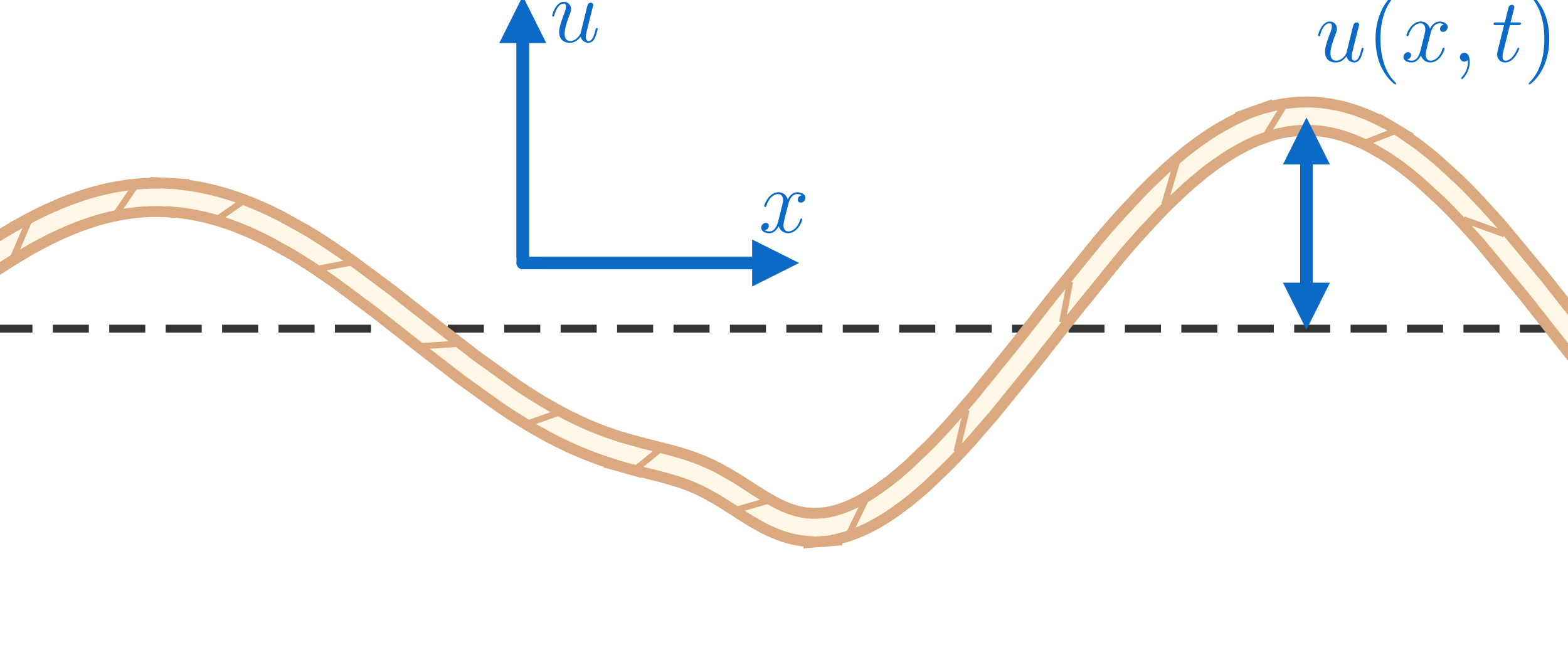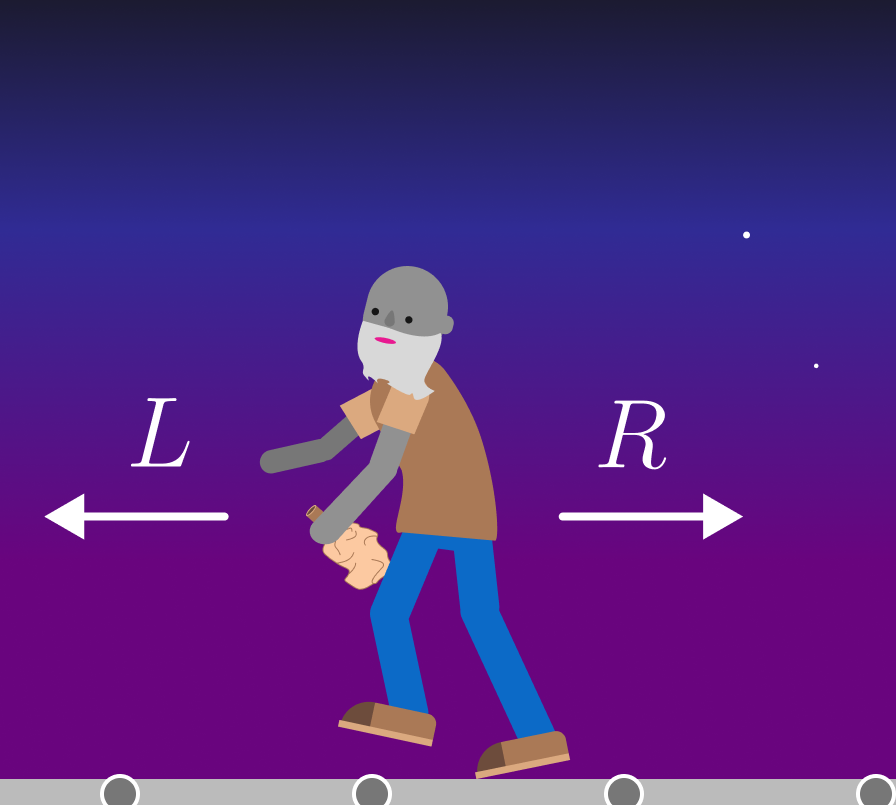### Differential Equations II

Besides being nonlinear, last quiz's equation systems had one other thing in common: their only independent variable was time $t.$

The other part of our course looks at problems where the situation is reversed: \begin{aligned} \textcolor{#D61F06}{\text{nonlinear section}} & \implies \textcolor{#D61F06}{\begin{cases} \text{several equations} \\ \text{single independent variable} \end{cases}} \\ \textcolor{#3D99F6}{\text{pde section}} & \implies \textcolor{#3D99F6}{\begin{cases} \text{single equation} \\ \text{several independent variables}. \end{cases}}\end{aligned} When the unknown function depends on several variables, it necessarily involves partial derivatives, hence the name partial differential equation, or PDE for short.

Let's take a look at some of the PDEs we'll encounter and how we'll go about solving them!

# PDEs in a Nutshell

You'll probably recognize our first PDE not from the equation itself but from its solutions:Tie a rope to a post and wiggle it up and down. The wiggles reflect back when they hit the post; the reflections and incoming wiggles combine and eventually form a standing wave.

The amount by which the rope is displaced depends on where we look and when, i.e. $u(x,t)$ (see figure) depends on $x$ and $t.$For reasons we'll get into later, the rope's wave equation is given by $u_{tt} = v^2 u_{xx},$ where $v$ is the constant wave speed and $u_{tt} \ (u_{xx})$ is the second partial derivative with respect to time (space).

Select all options solving this wave equation. Since we don't know how to solve a PDE from scratch yet, take the second partials of each and find those where $u_{tt}$ equals $v^2 u_{xx}.$

# PDEs in a Nutshell

Select one or more

The last problem not only shows us that a PDE can have more than one solution, but it also gives us a clue about how we can start making our own from scratch.

Notice that both solutions $\cos(v t) \sin(x)$ and $\sin(v t) \cos(x)$ “split” into $t$ and $x$ parts. Let's see if we can make a similar split $u(x,y,t) = X(x) Y(y) T(t)$ for the 2D wave equation $\frac{\partial^2 u}{\partial t^2 } = v^2 \left[ \frac{\partial^2 u}{\partial x^2 }+\frac{\partial^2 u}{\partial y^2 } \right],$ which describes the vibrations of the rectangular drumhead (blue) graphed below:

(The graph is touch interactive, so be sure to practice changing the perspective and zooming; we'll see many more graphs like this in the future!)

Plug $X(x) Y(y) T(t)$ into the 2D wave equation, and then divide both sides by $X(x) Y(y) T(t)$ after you're done taking derivatives. What can you say about the result?

# PDEs in a Nutshell

This method of variable separation is our first line of attack when it comes to PDEs. It won't always work, but when it does, it reduces a difficult PDE problem to something easier.

For example, the 2D wave equation splits as $\frac{T''(t)}{T(t)} = v^2 \left[ \frac{X''(x)}{X(x)} + \frac{Y''(y)}{Y(y)} \right];$ since $x,y,$ and $t$ are all independent variables, this equation can only be true if each individual piece is constant: $\frac{X''(x)}{X(x)} = - 4 \pi^2,\ \ \frac{Y''(y)}{Y(y)} = - 16 \pi^2,\ \ \frac{T''(t)}{T(t)} = -20 \pi^2 v^2$ are the choices that make the example defined on the rectangle $[0,1] \times \left[ 0, \frac{1}{2} \right]$ below.

Each of these equations can be solved separately; what option solves the $X$ equation?

# PDEs in a Nutshell

Waves on bounded domains like our drum are all infinite sums (called Fourier series) of “split” solutions like the ones we just found in the 2D case.

We'll also encounter another kind of infinite sum: power series. They're needed in a wide variety of real-world engineering and physics problems. Besides describing vibrations on a circular drumhead, they come up in fluid problems and even quantum theory.

In the finale of our course, we'll use power series together with separation of variables to solve the hydrogen atom, one of the most important scientific achievements of the 20$^\text{th}$ century.

We'll even use our solutions to sketch an iconic image from basic chemistry: an electron orbital!

# PDEs in a Nutshell

Separating the variables can be a useful thing to try when the domain has a simple shape like a rectangle or a circle, but even then we might need some advanced technique like power series.

Things are different for infinite domains, like that of the 3D compression wave we'll study later:

This wave obeys the 3D wave equation $u_{tt} = v^2 [ u_{xx}+ u_{yy}+u_{zz} ]$ or $u_{tt} = v^2 \nabla^2 u$ for short. $\big($The Laplacian $\nabla^2$ will be a recurring feature of our course!$\big)$

Here, $u(x,y,z,t)$ measures the compression/expansion of air at position $(x,y,z)$ at time $t.$

A 2D slice is shown below; to solve for $u,$ we'll need a new tool, the Fourier transform.

# PDEs in a Nutshell

The Fourier transform turns a PDE into an easier problem, like an ordinary differential equation. It works best when the domain is all of space, or $\mathbb{R}^{n},$ and the unknown $u$ “vanishes at infinity.”

For example, consider the classic Drunkard's walk problem in probability.

Jack's trying to make his way home after a night at the pub, but in his current state he moves left or right in a random way.If $x = 0$ is his starting point, then $u(x,t)$ measures the probability of locating Jack on the $x$-axis running along the sidewalk at time $t.$

It obeys the diffusion equation $\frac{\partial u}{\partial t} = \frac{\partial^2 u}{\partial x^2}\ \ \ \text{and} \ \int\limits_{x = - \infty}^{x=\infty} u(x,t) dx = 1,$ where the integral means we're certain to find Jack somewhere on the sidewalk at any given $t.$

Assuming the sidewalk is really really long, what “boundary conditions” do we need for this diffusion equation?

# PDEs in a Nutshell

Since $u \to 0$ as $|x| \to \infty,$ the diffusion equation is a perfect candidate for a Fourier transform.

The details will have to wait until later, but for now we can treat the transform $\mathcal{F}$ like a magic wand that turns a “$\frac{\partial}{\partial x}$” into a constant $i \omega = \sqrt{-1} \omega$ according to $\mathcal{F}\left[ \frac{\partial f}{\partial x} \right] = i \omega \mathcal{F}[f].$ Here, $\mathcal{F}[f]$ is called the Fourier transform of $f,$ which depends on $\omega$ now and not $x.$ For example, an important Fourier transform we'll prove later and use in the next problem is $\mathcal{F}\left[ A e^{-\frac{a x^2}{2}} \right] = \sqrt{\frac{1}{2 \pi a } } A e^{-\frac{\omega^2}{2a}}.$ If the Fourier transform doesn't affect $t$ at all, what differential equation does $\mathcal{F}[u]$ obey if $u$ satisfies the diffusion equation $u_{t} = u_{xx} ?$

# PDEs in a Nutshell

The Fourier transform really is magical: it turned the difficult diffusion problem $u_{t} = u_{xx}$ into the simpler first-order ordinary differential equation $\frac{d}{dt} \mathcal{F}[u] = - \omega^2 \mathcal{F}[u] \implies \mathcal{F}[u] =\text{(constant)} \cdot e^{-\omega^2 t}.$ But with all magic, there's a price: reversing the Fourier transform to get $u$ is normally tough.

Jack's random walk is an exception, though. Since we're certain he sets off from $x=0,$ $\mathcal{F}[u] = \frac{1}{2\pi} e^{-\omega^2 t};$ we'll work out the $\frac{1}{2 \pi}$ later. In the last problem, we quoted a specific Fourier transform: $\mathcal{F}\left[ A e^{-\frac{a x^2}{2}} \right] = \sqrt{\frac{1}{2 \pi a } } A e^{-\frac{\omega^2}{2a}}.$ Use this to find $u(x,t).$

# PDEs in a Nutshell

Jack's drunken stagger is a fun way to introduce the diffusion equation, one of the most important PDEs for many random processes appearing in physics, chemistry, and finance.

For example, atoms and molecules in a gas are jostled about unpredictably due to thermal motion, but a particle's trajectory is very much a 3D random walk with probabilities given by $u_t = \nabla^2u;$ $u(\vec{x},t)$ measures the likelihood it has diffused from its starting point to $\vec{x}$ in time $t.$

The Fourier transform can help us solve this higher-dimensional problem, too, but we first need to take the time to develop it and its inverse transform as triple integrals. More on this later!

# PDEs in a Nutshell

In a nutshell, a partial differential equation (PDE) has several independent variables.

There are many methods for approaching such an equation: separation of variables, power series, and the Fourier transform are just a few we touched on in this intro quiz.

Full mastery of PDEs and nonlinear equations takes time to develop, so let's begin!

# PDEs in a Nutshell

×